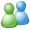Instant MessageSolution Library

## 1 Solution(s) found.

Question:  For the simple linear regression model, derive the estimates of the parameters. Using normal equations derive the estimates of constant and slope term